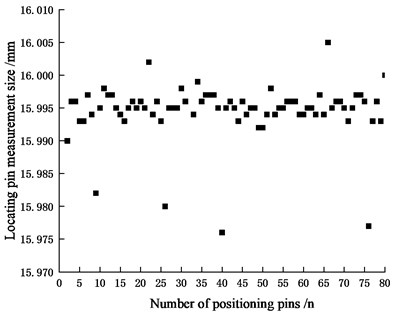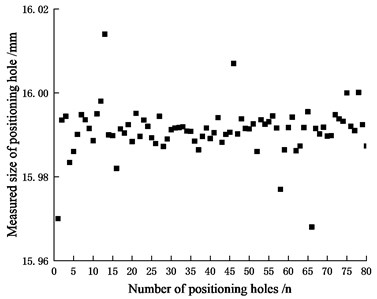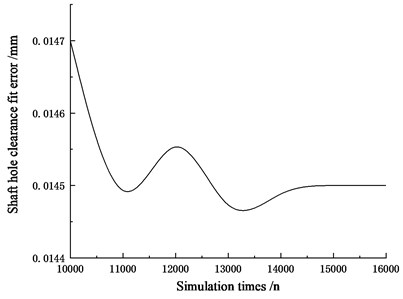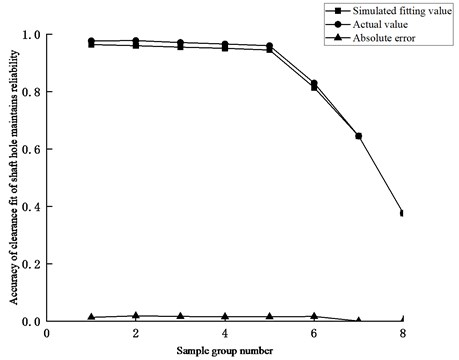Vibroengineering PROCEDIA

Published: 28 June 2021

# Analysis of uncertainty relation of pin-shaft fitting in mechanical system with clearance

Zhengkai Chu1
Dongfang Hu2
1, 2School of Mechatronics Engineering, Henan University of Science and Technology, Luoyang, 471003, China
2Collaborative Innovation Center of Machinery Equipment Advanced Manufacturing of Henan Province, Luoyang, 471003, China
Corresponding Author:
Zhengkai Chu
Views 42

#### Abstract

Pin-shaft fit is one of the most basic assembly structures for mechanical products, and the fit clearance plays a decisive role in the performance, safety and effective operation of the product. Taking the uncertainty of the shaft hole clearance fit in the positioning process as the research objective, based on the analysis of the factors affecting the uncertainty of the shaft hole clearance fit in the positioning process, an information entropy model of the shaft hole machining uncertainty is constructed to quantify describe the uncertainty of the shaft hole clearance fit. The uncertainty index of shaft hole clearance fit is proposed to comprehensively characterize the uncertainty of shaft hole clearance fit accuracy. And analyze the inherent relationship between the uncertainty index and the accuracy of shaft hole clearance fit keeping reliability. The effectiveness and feasibility of the method are verified through examples.

## 1. Introduction

Pin fit is one of the most basic assembly structures of mechanical products, and the fit clearance plays a decisive role in the performance, safety and effective operation of the product . Due to the existence of the assembly gap, the contact between the pin and the hole is random and uncertain. In order to ensure the machining accuracy of mechanical products, studying the uncertainty of the pin shaft during the clearance fit process has important engineering significance and application value .

Many scholars have conducted research in the field of reliability and uncertainty. Couto et al.  analyzed the influence of measurement uncertainty and proposed that a large value of measurement uncertainty often causes the actual process capability value to differ greatly from the observed value. Liu et al.  proposed a dynamic reliability model with mixed uncertain parameters in view of the randomness, interval and fuzziness of the parameters in the structural dynamic reliability analysis model, and discussed the effect of parameter types on reliability. Takeda  analyzed the random uncertainty in probabilistic risk assessment and developed a simple method based on the sensitivity coefficient, which can directly deal with the covariance of the parameters in the risk assessment, and compared it with the Monte Carlo method. The results are consistent. Ye et al.  proposed the uncertainty of the vibration performance of the bearing during service, and quantitatively analyzed the inherent specific relationship between the uncertainty index and the reliability of the vibration performance.

This paper takes the uncertainty of shaft hole clearance fit in the positioning process as the research objective. Based on the analysis of the factors affecting the uncertainty of shaft hole clearance fit in the positioning process, the uncertainty of shaft hole machining and the information entropy of contact uncertainty are constructed. The model is used to quantitatively describe the uncertainty of the shaft hole clearance fit error. It is proposed to use the shaft hole machining uncertainty and contact uncertainty to comprehensively characterize the uncertainty of the shaft hole clearance fit accuracy maintenance, and the uncertainty is analyzed. The internal relationship between the performance index and the accuracy of the clearance fit of the shaft hole to maintain the reliability.

## 2. Mathematical model

### 2.1. Processing uncertainty

The uncertainty of the contact point in the shaft-hole clearance fit is high, which is a key factor affecting the positioning error. How to measure their uncertainty is an important prerequisite for the control of shaft-hole clearance fit. In this paper, information entropy  is used to describe the uncertainty of shaft-hole clearance fit.

Assuming that in a sample, ${x}_{i}$ represents the actual size of part $X$, the probability of occurrence ${x}_{i}$ satisfies ${P}_{i}\ge 0$, $\sum _{i=1}^{m}{P}_{i}=1$, then the entropy of the assembly positioning error $\sum _{i=1}^{m}{P}_{i}=1$ is:

1
$H\left(X\right)=-K{P}_{i}\mathrm{l}\mathrm{n}{P}_{i}.$

The uncertainty of the actual size of the part $X$ can be defined as the entropy of the actual size of the part $X$, and its expression is:

2
$\mathbf{H}\left(\mathbf{X}\right)=\sum _{i=1}^{m}\mathbf{H}（{x}_{i}）=-K\sum _{i=1}^{m}{p}_{i}\mathrm{l}\mathrm{n}{p}_{i}.$

In a large sample, the entropy expression of the actual size of part $X$ is:

3
${H}_{X}=\int {H}_{X}dx=-K\int f\left(x\right)\mathrm{l}\mathrm{n}f\left(x\right)dx,$

where $K$ is a constant. $f\left(x\right)$ is the probability density function of $X$.

In a large sample, the actual size $x$ of the part $X$ obeys $N\left(\mu ,{\delta }^{2}\right)$, and 99 % of the actual size of the part is distributed in the interval $\left[\mu -3\delta ,\mu +3\delta \right]$, and its probability density function is $p\left(x\right)=\frac{1}{\sqrt{2\pi }\delta }\mathrm{e}\mathrm{x}\mathrm{p}\left(-\frac{\left(x-\mu {\right)}^{2}}{2{\delta }^{2}}\right)$.

In order to analyze the actual size entropy of the part and the theoretical size entropy of the part, since the shaft and hole process are consistent, the entropy change formula of the dissipative structure system is used for reference:

4
${S}_{1}=\sum _{i=1}^{n}\mathbf{H}\left({\mathbf{X}}_{i}\right)+C,$

where $C$ is a constant, ${S}_{1}$ represents the total entropy change of assembly production, and $\sum _{i=1}^{n}\mathbf{H}\left({\mathbf{X}}_{i}\right)$ represents the sum of the axis and hole information entropy.

### 2.2. Exposure uncertainty

Since there is a gap in the shaft hole during the fitting process, the contact of the shaft hole is completely random and uncertain. The Monte Carlo method is used to simulate the contact process of the shaft hole, and a large number of random numbers conforming to the overall distribution of the sample are randomly generated.

Assuming $P\left(A\right)$is the probability of occurrence of random event $A$, for the event that occurs $M$ times in the course of $N$ experiments, the frequency of occurrence of event $A$ is $f\left(A\right)=M/N$, and there is any real number $\epsilon >0$ that satisfies:

5
$\underset{x\to \infty }{\mathrm{l}\mathrm{i}\mathrm{m}}P\left(\left|\frac{M}{N}-P\left(A\right)\right|\le \epsilon \right)=1$

It can be seen from Eq. (7) that when $N$ is large enough, the probability of occurrence of event $A$ is $P\left(A\right)$. In addition, assuming that the independent and identically distributed variable is an $r$ random variable, the expected value is $\mathbf{E}\left({\mathbf{r}}_{k}\right)=\mathbf{\mu }$, and its variance is: $D\left({r}_{k}\right)={\sigma }^{2}\left({\sigma }^{2}\ne 0,k=1,2,3\cdots \right)$. Standardize the variables to obtain a new random variable as follows:

6
${\eta }_{n}=\frac{\sum _{k=1}^{n}{r}_{k}-E\left(\sum _{k=1}^{n}{r}_{k}\right)}{\sqrt{D\left(\sum _{k=1}^{n}{r}_{k}\right)}}=\frac{\sum _{k=1}^{n}{r}_{k}-n\eta }{\sqrt{n}\sigma }.$

Contact the data sample $X$, using the self-service gray method, randomly select 1 data from the data sample $X$ that can be replaced with a medium probability, and draw a total of $k$ times to obtain 1 self-service sample ${M}_{1}$ with $k$ data. Repeat this method $N$ times to get $N$ samples, which can be expressed as:

7
$\mathbf{M}=（{\mathbf{M}}_{1},{\mathbf{M}}_{2},\cdots ,{\mathbf{M}}_{n},\cdots ,{\mathbf{M}}_{N}）,$

where ${\mathbf{M}}_{n}$ is the $n$-th self-service sample, and $N$ is the total number of self-service re-sampling, which is also the number of self-service samples:

8

where $n$ is the sample serial number, $n=1,2,\cdots ,N$, and ${\mathbf{m}}_{n}\left(q\right)$ is the $q$-th self-service resampling data of ${\mathbf{M}}_{n}$.

The maximum entropy method is used to calculate the probability density function of the self-service resampling sample $M$. The lower limit value ${m}_{l}\left(k\right)$ and upper limit value ${m}_{u}\left(k\right)$ of the estimated interval $\left[{m}_{l}\left(k\right),{m}_{u}\left(k\right)\right]$ for the self-service calculation of the $k$-th contact state under the condition of the confidence level $P$ in the sampling sample $M$ should meet the following two conditions:

9
10
$\frac{1}{2}\left(1-P\right)={\int }_{{m}_{u}\left(k\right)}^{{m}_{k\mathrm{m}\mathrm{a}\mathrm{x}}}{f}_{k}\left(m\right)dv,$

where Eqs. (14) and (15), ${m}_{k\mathrm{m}\mathrm{i}\mathrm{n}}$ and ${m}_{k\mathrm{m}\mathrm{a}\mathrm{x}}$ are the minimum and maximum values in sample $M$, respectively.

Define the uncertainty of random contact as:

11
${S}_{2}=S\left(k\right)={m}_{u}\left(k\right)-{m}_{l}\left(k\right).$

### 2.3. Linear relationship analysis

According to the machining uncertainty index and the contact uncertainty index, the multi-factor regression analysis model of the shaft hole clearance fit error is established. The model function is:

12
$R={a}_{0}+{a}_{1}{S}_{1}+{a}_{2}{S}_{2}+{a}_{3}{S}_{1}{S}_{2}+{a}_{4}{{S}_{1}}^{2}+{a}_{5}{{S}_{2}}^{2},$

where ${a}_{0}$ is a constant term, ${a}_{1},{a}_{2},\cdots ,{a}_{5}$ is the corresponding coefficient of each variable.

For the judgment result of the multivariate regression analysis model, if the Durbin-Watson test statistic value is approximately equal to 2, it means that the residuals are independent. If the probability p value is less than the significance level, it also indirectly proves that there is a linear relationship between the shaft hole clearance fit error and the uncertainty index.

Observing the calculation results, if the ${a}_{4}$, ${a}_{5}$ coefficient is not 0, it indicates that the uncertainty index is the main non-linear influencing factor of the shaft hole clearance fit error. If the coefficient ${a}_{3}$ is not 0, it indicates that the interaction between uncertain indicators also affects the shaft hole clearance fit error.

## 3. Instance verification

### 3.1. Actual size of shaft hole

Taking a batch of cylindrical positioning pins with a diameter of 16 mm and a specification of h6 as the measurement object, the processed positioning pins are divided into 8 groups, 10 in each group, and each test piece is placed on the coordinate measuring machine for multiple times. The manual contact measurement method is used for the measurement, and the rigid probe is used, and the main body is used for axial scanning.

According to the measurement results, draw the size distribution diagram of the positioning pin. As shown in Fig. 1, it can be seen that the actual measured positioning pin diameter $d$ is basically distributed around 15.995 mm. The actual part size satisfies the 3$\sigma$ principle of part processing and conforms to the normal distribution law of mass production.

Fig. 1Locating pin measurement size distribution diagramTaking a certain batch of products with a diameter of 16 mm and a locating hole of H6 as the measurement object, the locating holes are divided into 8 groups, 10 in each group, and each test piece is placed on the coordinate measuring machine, and multiple measurements are obtained.

According to the measurement results, draw the size distribution diagram of the positioning hole. As shown in Fig. 2, it can be seen that the actual measurement positioning hole diameter d is basically distributed around 15.990 mm.

According to the shaft hole contact model, Monte Carlo method is used to generate the distribution function in line with the sample, and different simulation times are set. It can be seen from Fig. 3 that the shaft-hole clearance fit error is finally stabilized at 0.0145 mm. Compared with the theoretical error of the shaft-hole clearance fit, the shaft-hole fit is normal in the current state.

### 3.2. Multivariate regression analysis

For the specific quantitative relationship between the accuracy of the shaft hole clearance fit error retention reliability and the machining uncertainty and the shaft hole contact uncertainty, a multi-factor regression analysis model needs to be established. The model function is:

$R={a}_{0}+{a}_{1}{S}_{1}+{a}_{2}{S}_{2}+{a}_{3}{S}_{1}{S}_{2}+{a}_{4}{{S}_{1}}^{2}+{a}_{5}{{S}_{2}}^{2},$

where ${a}_{0}$ is the constant term. The constant term and various coefficient values in the model function are: ${a}_{0}=$ 1.322, ${a}_{1}=$ –0.168, ${a}_{2}=$ –0.273, ${a}_{3}=$ 0.142, ${a}_{4}$ = 0.018, ${a}_{5}$ = –0.003.

Fig. 2Positioning holes measurement size distribution diagramFig. 3Based on Monte Carlo simulation of shaft hole clearance fit error distribution diagramIn the fitting results, the multiple correlation coefficient of the model is 0.985, the determination coefficient is 0.977, the adjustment determination coefficient is 0.028, and the test statistic is 1.794, its value is close to 2, and the residuals are independent. In the results of the analysis of variance of the model, the F statistic value is 34.841, the probability $p$ value is 0.022, and the significance level is lower than 0.050. Therefore, it is considered that there is a linear relationship between the accuracy of the shaft hole clearance fit error and the uncertainty index.

The fitting effect of the multivariate regression analysis model is shown in Fig. 4.

Fig. 4Fitting effect of multivariate regression analysis modelThe results show that the maximum absolute value of the standardized residuals of the regression model is 0.964, which does not exceed the default value of 3, so it can be considered that there is no singular value. ${a}_{4}$, ${a}_{5}$ are not 0, indicating that the machining uncertainty ${S}_{1}$ and contact uncertainty ${S}_{2}$ are the main non-linear influencing factors of the shaft hole clearance error. At the same time, ${a}_{3}$ is not 0, indicating that the interaction between the machining uncertainty ${S}_{1}$ and the contact uncertainty ${S}_{2}$ also affects the accuracy of the shaft hole clearance fit error accuracy maintenance reliability.

## 4. Conclusions

1) The information entropy model of shaft hole machining uncertainty and contact uncertainty is constructed to quantitatively describe the uncertainty of shaft hole clearance fit error. An uncertainty index is proposed to comprehensively characterize the uncertainty of the accuracy of the shaft hole clearance fit error, and the internal relationship between the uncertainty index and the accuracy of the shaft hole clearance fit error accuracy maintenance reliability is analyzed.

2) The results of multi-factor regression analysis show that machining uncertainty ${S}_{1}$ and ${S}_{2}$ contact uncertainty are the main non-linear influencing factors of the shaft hole clearance error.

#### Acknowledgements

The research was supported by the National Natural Science Foundation of China (Project No. 51775172) and the Key Scientific Research Projects of Colleges and Universities in Henan Province (Project No. 19A460020).